##### Geometry Workbook For DummiesProving that a quadrilateral is a kite is a piece of cake. Usually, all you have to do is use congruent triangles or isosceles triangles. Here are the two methods:

• If two disjoint pairs of consecutive sides of a quadrilateral are congruent, then it’s a kite (reverse of the kite definition).

• If one of the diagonals of a quadrilateral is the perpendicular bisector of the other, then it’s a kite (converse of a property).

When you’re trying to prove that a quadrilateral is a kite, the following tips may come in handy:

• Check the diagram for congruent triangles. Don’t fail to spot triangles that look congruent and to consider how CPCTC (Corresponding Parts of Congruent Triangles are Congruent) might help you.

• Keep the first equidistance theorem in mind (which you might use in addition to or instead of proving triangles congruent): If two points are each (one at a time) equidistant from the endpoints of a segment, then those points determine the perpendicular bisector of the segment. (Here’s an easy way to think about it: If you have two pairs of congruent segments, then there’s a perpendicular bisector.)

• Draw in diagonals. One of the methods for proving that a quadrilateral is a kite involves diagonals, so if the diagram lacks either of the kite’s two diagonals, try drawing in one or both of them.

Now get ready for a proof: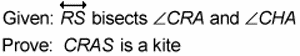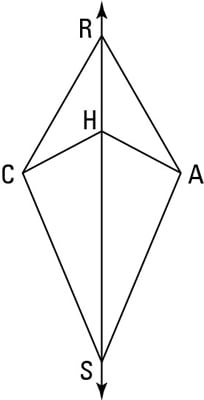Game plan: Here’s how your plan of attack might work for this proof.

• Note that one of the kite’s diagonals is missing. Draw in the missing diagonal, segment CA.

• Check the diagram for congruent triangles. After drawing in segment CA, there are six pairs of congruent triangles. The two triangles most likely to help you are triangles CRH and ARH.

• Prove the triangles congruent. You can use ASA (the Angle-Side-Angle theorem).

• Use the equidistance theorem.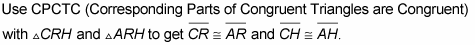Then, using the equidistance theorem, those two pairs of congruent sides determine the perpendicular bisector of the diagonal you drew in. Over and out.

Check out the formal proof:

Statement 1: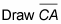Reason for statement 1: Two points determine a line.

Statement 2: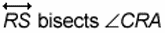Reason for statement 2: Given.

Statement 3: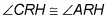Reason for statement 3: Definition of bisect.

Statement 4: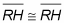Reason for statement 4: Reflexive Property.

Statement 5: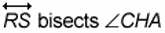Reason for statement 5: Given.

Statement 6: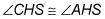Reason for statement 6: Definition of bisect.

Statement 7: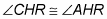Reason for statement 7: If two angles are supplementary to two other congruent angles (angle CHS and angle AHS), then they’re congruent.

Statement 8: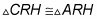Reason for statement 8: ASA (3, 4, 7).

Statement 9: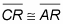Reason for statement 9: CPCTC.

Statement 10: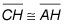Reason for statement 10: CPCTC.

Statement 11: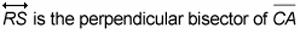Reason for statement 11: If two points (R and H) are each equidistant from the endpoints of a segment (segment CA), then they determine the perpendicular bisector of that segment.

Statement 12: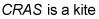Reason for statement 12: If one of the diagonals of a quadrilateral (segment RS) is the perpendicular bisector of the other (segment CA), then the quadrilateral is a kite.